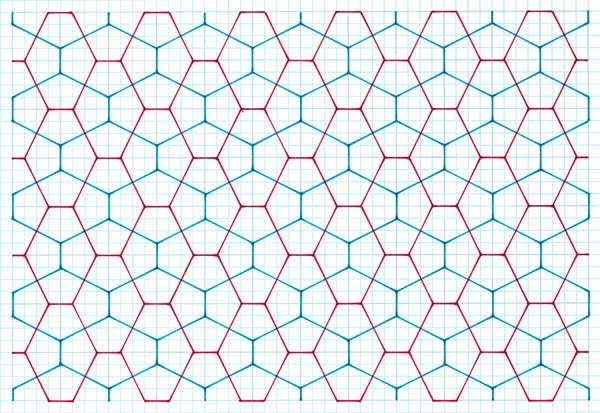Cairo Tiling‎ > ‎

### Study 1

 Stick Cross MethodThe particular study consists of arguably the most simplest possible constructions for a Cairo-like tiling, namely that of a stick cross, of two equal arms, possessing order 4 rotational symmetry, placed in a square matrix, and then successively reflected. The study is presented in a series of three stages: 1 shows the premise, 2 shows the tiles arising from this as tilings, 3 gives comment on the findings, effectively as a summary.PremiseFigure 1: Reflected Stick cross principleThe premise of this particular construction is one of undoubted simplicity, of symmetrical stick crosses reflected in a square matrix, as according to unit size intersections; the example above based on a 3 x 3 unit, Figure 1. Two different coloured lines that show the ‘reflected placement’ premise. As can be seen this, this basic procedure can be varied and so make for considerable differences of the cross, in terms of its ‘angularity’, and scale. The cross can vary in its ‘angularity of placement’ as according to the underlying intersections of the unit square used and by increasing the unit size of the square, Figure 2.Figure 2: All Possibilities of Stick CrossesFigure 3: A Set of 15 Distinct TilesAs can be seen the proportions of the resulting pentagons can be seen to differ markedly, from a ‘near’ rectangle (13) and ‘near’ square (11), of which rectangles and squares is indeed obtained if the stick cross is placed at the minimum and maximum points. Nonetheless, despite such extremes, all the while the tiles still retain their ‘Cairo-type’ tiling properties as previously detailed. Tilings Having thus determined distinct individual tiles, these are thus shown as tilings, Figure 4. Note that for the sake of a more concise presentation, I show only the first five examples of the sequence, followed by some examples that are of ‘of special interest’.Figure 4: Angles of 108.43°, 143.13°Figure 5: Angles of 116.57°, 126.8°Figure 6: Angles of 120.6°, 118.07°Figure 7: Angles of 101.31°, 157.38°Figure 8: Angles of 123.69°, 112.62°Figure 9: Angles of 113.2°, 133.6°Figure 10: Angles of 126.87°, 106.26°CommentsAs can be seen, when shown as a tiling this particular premise always results in a single identical pentagon, and so being a minimum number is obviously ‘aesthetic’ (as I later show with other, different premises, is not always so. Obviously, 2, 3, 4 or more pentagons in a composition lack this (of which I show in succeeding studies), as interesting as they (or can be) are. The subsidiary hexagons are at right angles, which again is aesthetically pleasing, and as above, this is not necessarily so with others.Of interest are the proportions of the pentagons, which vary considerably, from ‘near’ square to ‘near’ rectangle, at least in relative terms (certainly, with other studies, the connection is much clearer). Aesthetically, these ‘extreme’ examples are lacking, in that these are furthest away from an ‘average’ pentagon.Of interest is to compare the pentagons so discerned with those of the ‘aesthetic’ type frequently cited (although without in situ evidence), namely the equilateral and dual types, and see how close these can come to such ‘aesthetic’ examples, and indeed of the in-situ pentagons themselves: Equilateral 114° 18’ (2) and 131° 24’Tiling 7/15, with angles of 113.2° (2), and 133.6° is close. 32.4.3.4 120° (3)3/15 is very close, with almost alike angles (120.96°, 118.07°).In situ pentagonsAngles of 108.43°, 143.13°A simple observation is that these are collinear to the ‘nearest neighbour’, which thus has implications on this. Of note is that the only square-based example with the same condition is 1/15.Created 31 August 2011. Updated 14 July 2020. Removed the now outdated side length ratio measures e.g.  2: 1.58 and outdated angle notation. The 'odd' ratio format was forced upon me by GeoGebra by my drawing process, which began with a unit of 2, instead of 1! The angles were measured in GeoGebra, but to which I was under the impression that these were of degrees and minutes, rather than decimals. e.g. 108°.43’, 143.13 should be 108.43, 143.13 in decimal!